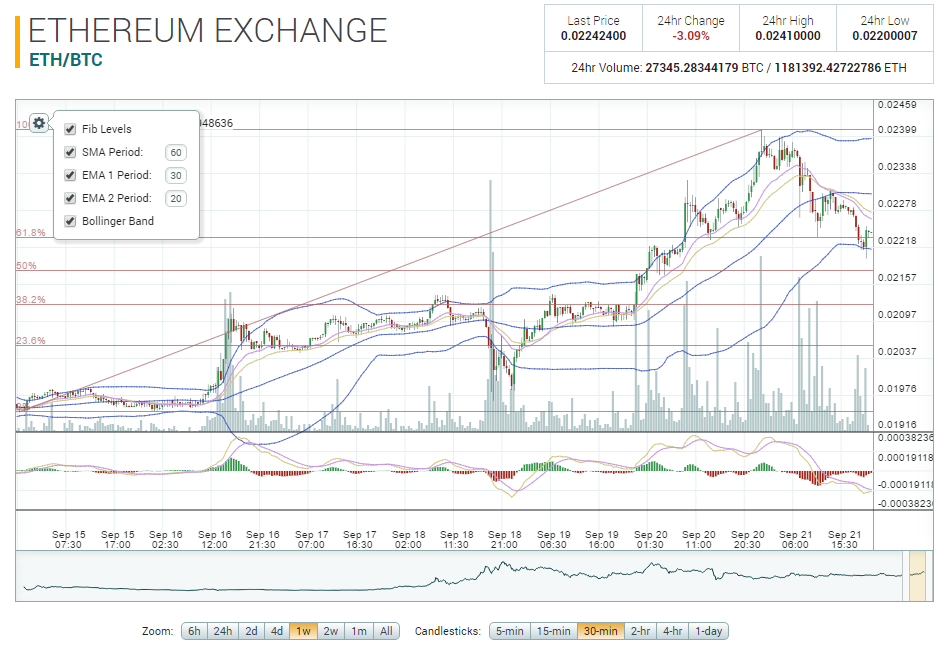##### About PoloniexBased in the United States, Poloniex is a leading cryptocurrency exchange offering over 100 Bitcoin (BTC) markets available for trading. Founded in January 2014, Poloniex offers a secure trading environment and provides advanced charts and data analysis tools to its customers.

if you are trade in poloniex, you will see the tools (indicator) in the charts. its will help for start trading, when the best make open buy or sell an altcoin. let me introducing the indicator on poloniex...

ok, for the first..

what is INDICATORS ??

Technical Indicators are the often squiggly lines found above, below and on-top-of the price information on a technical chart. Indicators that use the same scale as prices are typically plotted on top of the price bars and are therefore referred to as âOverlaysâ.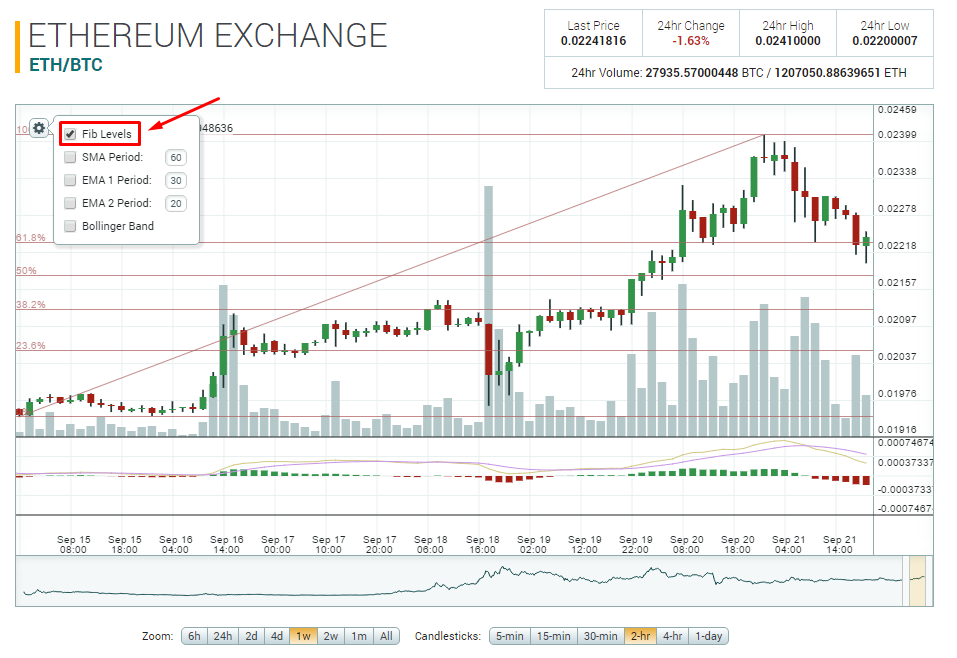1. fib levels or Fibonacci Retracement
Fibonacci tool is that it works best when the market is trending.
The idea is to go long (buy) on a retracement at a Fibonacci support level when the market is trending up, and to go short (sell) on a retracement at a Fibonacci resistance level when the market is trending down.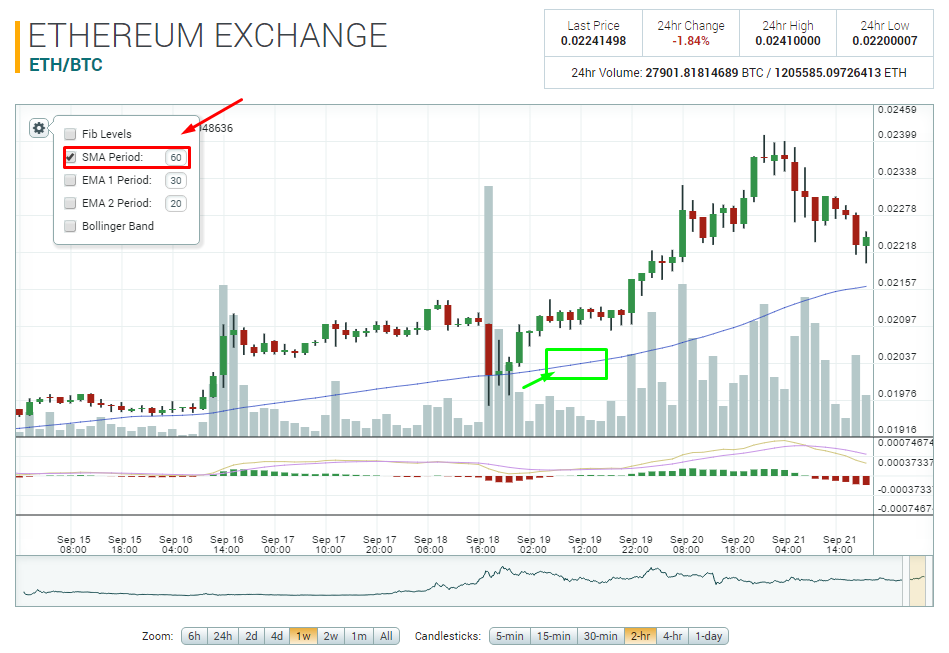2. SMA or Simple Moving Average
A simple moving average (SMA) is the simplest type of moving average in forex analysis. Basically, a simple moving average is calculated by adding up the last âXâ periodâs closing prices and then dividing that number by X.
##### use the default or following the basic tool (how those it work).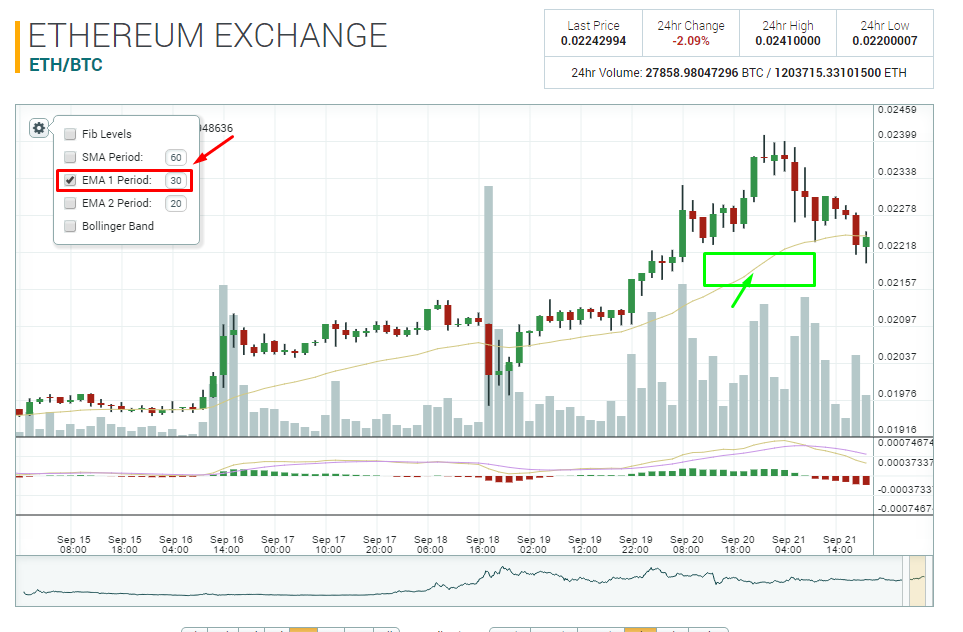3. EMA (include ema 1 and 2) or Exponential Moving Average
An exponential moving average (EMA) is a type of moving average that is similar to a simple moving average, except that more weight is given to the latest data. It's also known as the exponentially weighted moving average. This type of moving average reacts faster to recent price changes than a simple moving average.
##### use the default or following the basic tool (how those it work).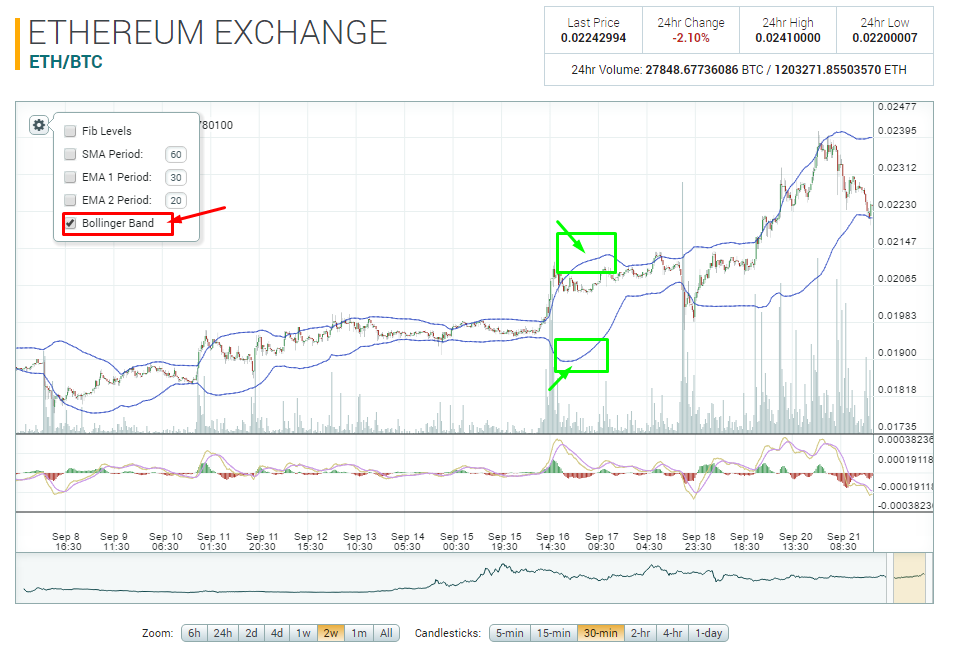3. bollinger band
Bollinger Bands consist of a middle band with two outer bands. The middle band is a simple moving average that is usually set at 20 periods. A simple moving average is used because the standard deviation formula also uses a simple moving average. The look-back period for the standard deviation is the same as for the simple moving average. The outer bands are usually set 2 standard deviations above and below the middle band.

SharpCharts Calculation
*Middle Band = 20-day simple moving average (SMA)
* Upper Band = 20-day SMA + (20-day standard deviation of price x 2)
* Lower Band = 20-day SMA - (20-day standard deviation of price x 2)

DONE !!

note :
- this post just introdution chart tool/indicator-litle explanation. for details, you can search on google.
it will spending all page if explaining here. and i'm just newbie trader :D